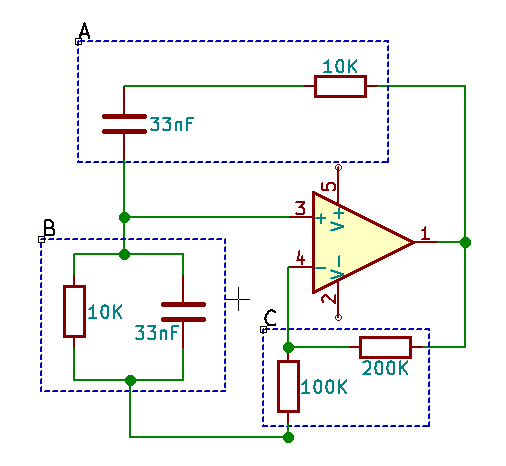## Sine Wave Generator

PSLab by FOSSASIA can generate sine waves with arbitrary frequencies. This is very helpful to teachers, students and electronic enthusiasts to study about different frequencies and how systems respond to them. In the device, it uses digital signal processing to derive a smooth sine wave. Except to digital implementation, there are conventional analog implementations to generate a sine wave.The most famous method to generate a sine wave is the Wien Bridge Oscillator. It is a frequency selective bridge with a range of arbitrary frequencies. This oscillator has a good stability when it is functioning at its resonance frequency while maintaining a very low signal distortion.Let’s take a look at this circuit. We can clearly see that there is a series combination of a resistor and a capacitor at A and a parallel combination of a resistor and a capacitor at B joining at the non-inverting pin of the OpAmp. The series combination of RC circuit is nothing but a high pass filter that allows only high frequency components to pass through. The parallel combination of RC circuit is a Low pass filter that allows only the low frequency components of a signal to pass through. Once these two are combined, a band pass filter is created allowing only a specific frequency component to pass through.

It is necessary that the resistor value and the capacitor values should be the same in order to have better performance without any distortion. Assuming that they are same, using complex algebra we can prove that the voltage at V+(3) is one third of the Voltage coming out from the pin (1) of OpAmp.

Using the resonance frequency calculation using RC values, we can determine the frequency of the output sine wave.

f=1/2RC

The combination of two resistors at the inverting pin of the Op Amp controls the gain factor. It is calculated as 1+R1/R2. Since the input to the non-inverting terminal is 1/3 of the output voltage, this gain factor should be maintained at 3. Values greater than 3 will cause a ramping behavior in the sine wave and values below 3 will show an attenuation. So the gain should be set preciously.

Equating 1+R1/R2 to 3, we can obtain a ratio for R1/R2 as 2. That implies R1 should be as twice the resistance of R2. Make sure these resistances are in the power of kilo Ohms. That is to ensure that the leakage current is minimum to the Op Amp. Let’s select R1 = 200K and R2 = 100K

This oscillator supports a range of frequencies. Let’s assume we want to generate a sine wave having 500 Hz. Using f=1/2RC, we can choose arbitrary values for R and C. Substituting values to the formula yields a value for RC = 318 x10e-6Using practical values for R as 10k, C value can be approximated to 33nF. This oscillator is capable of generating a stable 500 Hz sinusoidal waveform. By changing the resistive and capacitive values of block A and B, we can generate a wide range of frequencies that are supported by the Op Amp because Op Amps have a limited bandwidth it can be functional inside.

It’s worth to note a few facts about generating sine waves using a digital implementation. In analog circuitry, the component values have a tolerance which makes the calculations not perfectly align with the real values. Due to this reason, the actual output will differ from the desired output. In our example to generate 500 Hz sine wave, the capacitors and resistors may have different values and they may have not matched. But with a digital implementation, we can achieve the accuracy and flexibility we require.